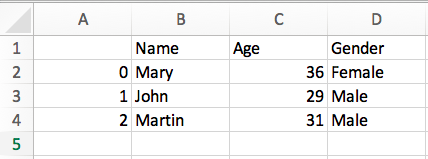# Python Archive#### How to initialize array in Python

In this article, we will see a different ways to initialize an array in Python. Array is collection of elements ...#### How to print Array in Python

In this post, we will see how to print array in Python. As we know that, Python didn't have an ...#### Remove all instances of element from list in Python

Learn about different ways to remove all occurrences or instances of a given element from the list in Python ...#### Reorder the columns of pandas dataframe in Python

In this post, we will see 3 different methods to Reordering the columns of Pandas Dataframe : Using reindex method ...#### Pandas create Dataframe from Dictionary

In this tutorial, We will see different ways of Creating a pandas Dataframe from Dictionary . Using a Dataframe() method ...#### Pandas Convert list to DataFrame

In this tutorial, We will see different ways of Creating a pandas Dataframe from List. You can use Dataframe() method ...#### Python | Introduction and Installation of OpenCv

In this tutorial, we are learning about what is OpenCv and how OpenCV is installed in windows/linux system. Introduction Have ...#### Python | cv2 imshow() Method

In this tutorial, we will see how to display an image as an output using python by the use of ...#### Python | cv2 imwrite() Method

In this tutorial, we will see how to save an image in your own system using python by using open-cv ...#### Pandas DataFrame to CSV

In this post, we will see how to save DataFrame to a CSV file in Python pandas. You can use ...